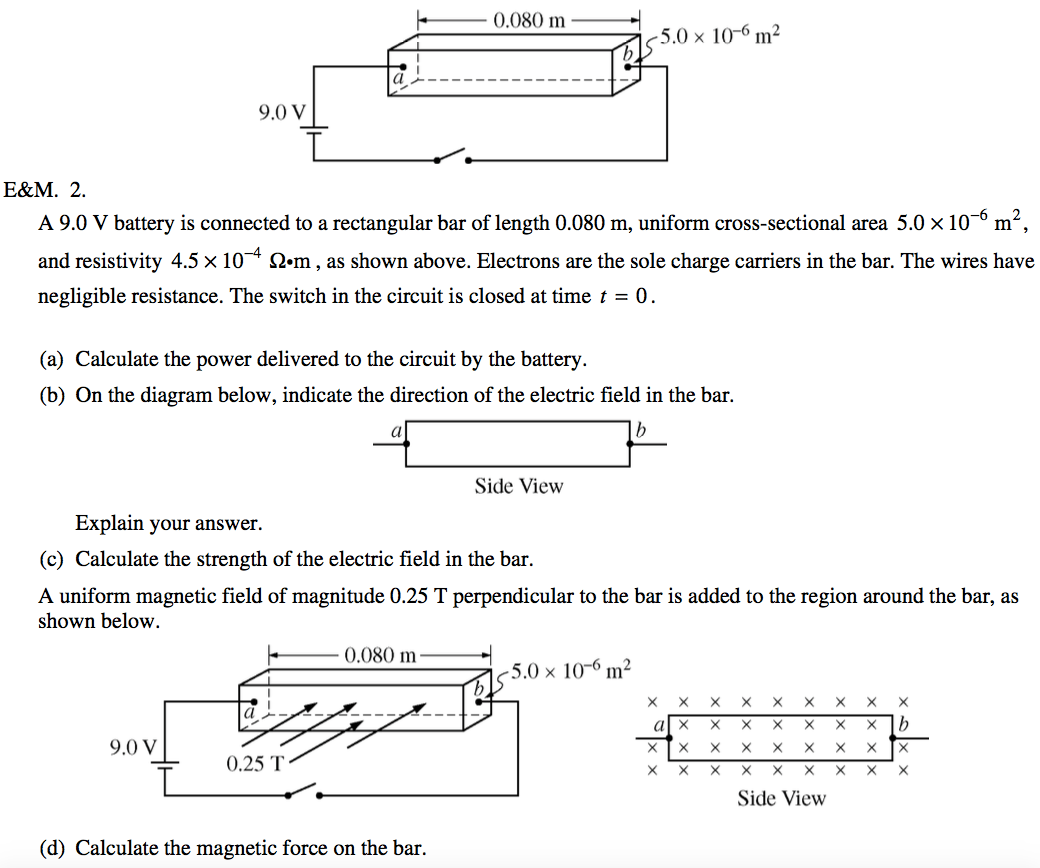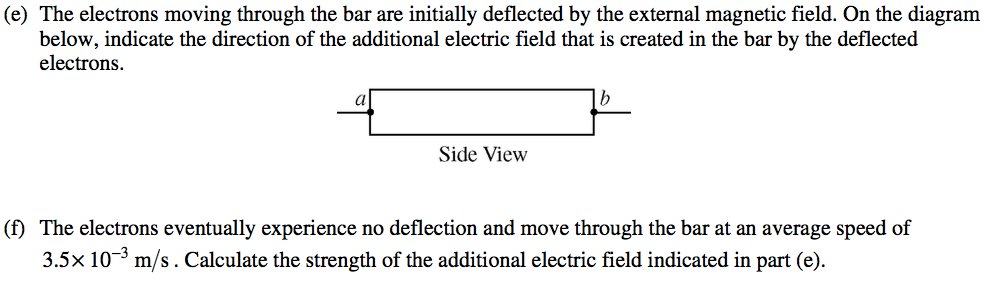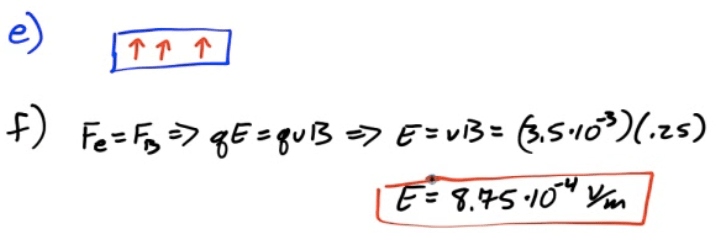# Example 1: Torque on a Loop of Wire

• A loop of wire carrying current I is placed in a magnetic field. Determine the net torque around the axis of rotation due to the current in the wire.# Magnetic Field due to a Current-Carrying Wire

• Moving charges create magnetic fields

• Current-carrying wires carry moving charges, therefore they create B fields

• Direction given by right-hand rule

• For multiple wires, determine B field from each and add them up using superposition

• B fields may interact with other moving charges, so current-carrying wires can exert forces upon each other.

# First Right Hand Rule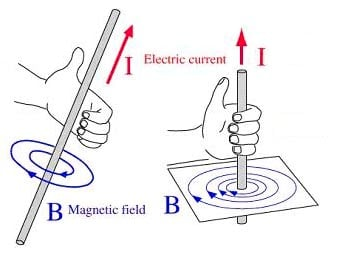• "Hold" wire with your right hand, thumb in direction of positive current flow

• Your fingers wrap in the direction of the magnetic field

•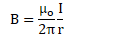•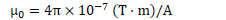# Example 2: Magnetic Field due to a Wire

• A wire carries a current of 6 amperes to the left.

• Find the magnetic field at point P, located 0.1 meters below the wire# Force Between Parallel Current-Carrying Wires

• Use right hand rules to determine force between parallel current-carrying wires

• Find magnetic field due to first wire. Draw it.

• Find direction of force on second wire due to current in second wire. Force on the first wire will be equal and opposite (Newton's third Law)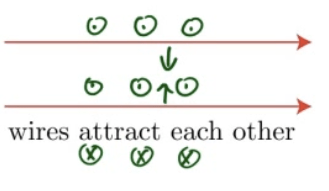# Gauss's Law for Magnetism

• You can never draw a closed surface with any net magnetic flux because there are no magnetic monopoles.

• This is the basis of Gauss's Law for Magnetism (Maxwell's second equation)

•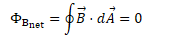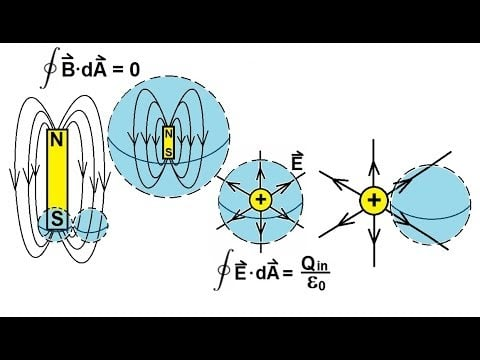# Example 3: Field due to Wires

• Two long current-carrying wires are separated by a distance d as shown.

• What is the net magnetic field due to these wires at point P, located midway between the two wires, if the top wire carries a current of 3A and the bottom wire caries a current of 5A.

•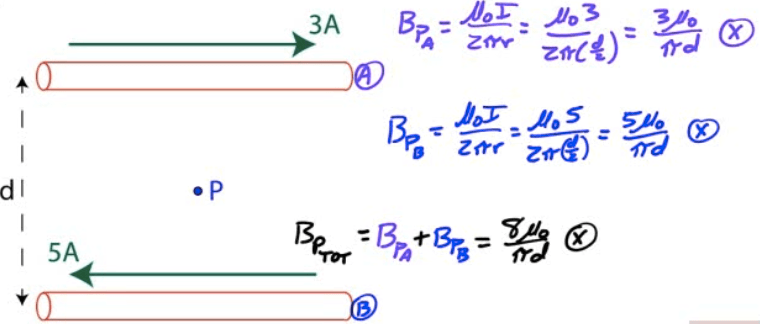# Example 4: Force on a Wire

• A 5-m long straight wire runs at a 45-degree angle to a uniform magnetic field of 5 T. If the force on the wire is 1N, determine the current in the wire

••# 2009 Free Response Question 2